Mathematics Made Easy by N. S. Murty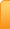Channels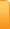Analysis
Cartoons
Education
Environment
Opinion
Photo Essays

Random Thoughts

Architecture
Astrology
Ayurveda
Buddhism
Cinema
Culture
Festivals
Hinduism
History
People
Places
Sikhism
Spirituality

Health
Parenting
Perspective
Recipes
Society
Teens
Women

Creative Writings

Book Reviews
Computing
Humor
Individuality
Literary Shelf
Memoirs
Quotes
Stories
Travelogues
Workshop

by N. S. Murty

When I was a child I heard mathematics was tough and hard and even without attempting it, I started fearing it. I don’t exactly remember how this fear was allayed by my teacher, but one fine morning I started doing math on my own and slowly it developed into a passion. I am sure most of the children experience(d) the same way I did and I just want give few important tips from our old Vedic tradition. I don’t try to give you any Sanskrit Sutras but shall give you the essence of it in a capsule. This ranges from easy methods of multiplication, squaring, finding the products of large numbers, solutions to quadratic, simultaneous equations by mere observation etc. All these things one can do without using a calculator. If one develops the skill by constant practice, I am sure anyone can do these most of the times by mere observation and simple mental calculation.

I start with a sample of finding the squares of small numbers orally (and later expand it to finding tables upto 20X20 just using the ten fingers of both hands.)

First finding the square: ( I am confining myself to numbers up to 20 only for the present and as you shall see it can be extended to any number later)

The rule is as follows

When you are given a number to find its square, take ten as the base, and if the number is less than (more than) ten, then subtract (add) by the amount it was less (or more) from (to) the given number. Treat it as tens. Then simply square the number you subtracted from ten (or added to ten) and put it as units. If the square of this number has tens and units, carry over the tens to the tens we have already.

Explanation by example

a)
Let us suppose we are given to find out the square of the number 8
Taking 10 as base, 8 is less than ten by 2.
So subtract 2 from 8. You get 6. Put it in tens place. Then square 2 (8 is less than 10 by 2) which is 4 and put it in units. So the square of 8 is 64.

b)
Let us suppose we have to find the square of 12.
12 is more than ten by 2. So add 2 to 12. We get 14. Put it in tens.
Then square 2. We get 4. Put it in units place. We thus get 144 as the square of 12.

c)
Find the square of 6.
6 is less than 10 by 4. subtract 4 from 6 and put it in tens. We have 2 tens.
Then square 4 and we get 16 as its square. This has tens and units. Carry the tens to the tens we already have. Thus we get 2 + 1 = 3 tens and 6 units. So square of 6 is 36.

d)
Find the square of 16
16 is greater than ten by 6. So add another 6 to 16. We get 22 and put it under tens.
Square 6. We have 36. Carry 3 to tens and put 6 in units. We have 22+3 = 25 tens and 6 units. So 16 square is 256.

e)
Find the square of 19.
19 is more than 10 by 9. Add another 9 to 19. We get 28 and put it in tens.
Square 9 and we get 81. Carry 8 to tens and keep 1 in units. Then we get 28+8 = 36 tens and 1 unit which is 361. So square of 19 is 361.

It is so easy. Isn’t it? Now you should be able to tell the square of any number between 1 and 20 without committing it to memory.

II

You must have noticed when the number is above 15 and when you take 10 as base the calculation is somewhat difficult unless you have mastered the squares up to 9.

Now we have another rather easy method.

When the given number is nearer to a 10 multiple, take that 10 multiple as the base and find the square but, with a little adjustment for the new base. Thus you will find it easy to take 20 as base instead of 10 when you need to calculate the squares of numbers above 15. We have already seen when we take 10 as base we are subtracting from or adding to the given number the quantity by which it differs from 10 and putting as tens.

Similarly, when we take 20 or 30 or 70 as base, we will be getting values in 20s, 30s, and 70s. So we must multiply these 20s, 30s, and 70s appropriately by 2, 3 and 7 to convert them to 10s. That is all the adjustment we need to make. Let us see by example.

Let us calculate the square of 19.

Take 20 as base. Then 19 is less than 20 by 1. So subtract 1 from 19 and put it as 20s. So we get 18 twenties and multiplying it with 2 we get our 10s. Thus we have (19-1)= 18 twenties which is 36 tens. As usual we square the 1 and we get 361 which is the square of 19. You can see by taking 10 as base we got 19+9 =28 tens and another 8 tens from the square of 9 making 36 tens. It is likely that we forget to add these tens from 9 square. So it is always better to take the 10 multiple nearer to the given number.

Let us find the square of 39.

Take 40 as base. We get 39-1= 38 forties or 38x4= 152 tens. Answer is 1521. This you can say in a trice without multiplying by the usual long drawn method. Isn't it?

Try 78 square.

Take 80 as base. 78 is less than 80 by 2. You get 78-2 =76 eighties or 76x8 = 608 tens hence 78 square is 6084

Try 44 square

Take 40 as base. 44 is 4 greater than 40. So we get 44+4 = 48 forties or 192 tens. Squaring 4 we get another 1 ten and 6 units. Answer therefore is, 1936.

Thus you can find the square of any number between 1 to100 now. Can't you?
Well I know you ask if the number is like 25, 35, 45, 65, etc exactly in the middle of twenties, thirties etc., you do not see advantage of one method over the other. But strangely, you don't need any of these two methods at all. There is nothing simpler than such numbers having 5 in the units place to find the square for. It is the easiest of all things.

All you have to do is take the number to the left of 5 and multiply it by its next number and put the product in 100s and always put 25 to the right of it. Just that.

Let us see by example. Find the square of 25. Now the number to the left of 5 is 2. The next number to 2 is 3. Then 2x3 is 6 we got 6 hundreds. (I am talking about the place value) Putting 25 to the right of it we get 625.

35 square is 3x4= 12 therefore 1225
45 square is 4x5=20 therefore 2025
75 square is 7x8= 56 therefore 5625
95 square is 9x10=90 therefore 9025 and so on.

Now I am sure you can say what is the square of 125.

Yes.

12x 13 = 156 Therefore 125 square is just 15625.

How much time did you take to answer this?
There is a very interesting corollary, that means, which follows this operation.
For any two numbers between the sets of ten like 21-29, 31-39, 41-49 , ... 219-220.551-559...so on,

When the sum of the numbers in the units place is ten, then also the above principle holds. That is multiply the number to the left of units place by its next number and put it in hundreds' place. However the difference now is instead of 25 you will put the product of the two numbers in the units place.

Let us study by example:

Find the product of 23 and 27.

Since 3+7 is ten, the value is 621 See the following table:

21 * 29 it is 609
(don't forget that when there is no value for tens, there is 0 always.)
22 * 28 it is 616
23 * 27 it is 621
24 * 26 it is 624
25 * 25 it is 625
82 * 88 it is 7216 ( remember the carry forwards)
121 * 129 is 15609
124 * 126 it is 15624

Thus it is fun to find the products of numbers.

III

Now let us move a bit further and find out the products of different numbers.

Suppose you need to multiply 95 and 97 or 51 and 59 or 35 and 39 etc. We have a wonderful system in place which helps us find the product of any pair of multiplicands.

The rule says that whenever you come across pairs of multiplicands like above, write the multiplicands one below the other and follow the procedure detailed below:

Step 1: Take the nearest multiple of 10 as base

Step 2: Subtract the base from the multiplicands individually and put the value with sign to their right.

Step 3: Cross add the multiplicands and the differences check they are equal. When the base is 10 to 90, this value represents 10s or 90s as the case may be.

Step 4: Multiply the differences and put them in the units place. (It goes without saying that whenever the product exceeds ten there will be carry over of 1)

Yes. The process looks so long and cumbersome. But in reality it is damn easy and has a self-check for the value obtained.

Let us see. The given example is to fine the product of 95 and 97. By observation we can take 100 as base.

Put 95 and 97 one over the other

95
97

The difference of the numbers with base looks like this:

95 -05
97 -03

You can see by cross adding the multiplicands and the differences, here 97-5=92 as also 95-3=92. Since we have taken 100 as base what we got this value is 100s. Then multiplying (-5) and (-3) we get 15. Thus the product of 95 and 97 is 9215.

There is another interesting cross check. You add 95 and 97 and subtract 100 from it. You get 92. You add 5 and 3 the differences and subtract from 100, you still get 92. Thus when we take any base, remember clearly that the value we get in these operations is ‘that many times’ the base we have chosen.

So when you remember it you will make necessary adjustments to get 10s or 100s. Let us go to the second example: The process reads as follows:

Take 50 as base. Write the given numbers 51 and 59 as follows:

51 +1
59 +9
---------
60    9
---------

and as already explained the 60 on the left side is 50s as such the real value in 10s is 60X50= 3000 or 30 100s by adjustment. So the final value is 3000+ 09= 3009

Let us see one more: 35X39

Take 40 as base by observation. The procedure looks like this:

35 -5
39 -1
----------
34    5
----------

Since the value 34 represents 40s the value in tens is 34X4 = 136. So the final value is 1365

Am I clear?

Let us recap what we did and what our operations mean. Whenever we are subtracting the base from the given number, we are trying to find out how far is our number from the base. Thus when two such numbers are multiplied, we are getting the shortage from the product of the bases. But in the number system, this product place is nothing but the x 2 term. That is why when we take 50 as base we are getting 50s and 40 as base 40s. When we take 100 as base, as in the first case for example, we got 100s directly. Because we are working with the decimal system and our bases are not 10 or 100 but different numbers for operational convenience, we are making necessary adjustments to get back to decimal system. You can verify the old examples by this method as well.

Base: 90

82    -8
88     -2
---------

80 +16

Here base being 90, the value is (90X8 =720 in tens or 72 in hundreds) 7216

Base: 120

121 +1
129 +9
---------

130    9

Since 120 is the base here the final value is (13X12 = 156 in hundreds) 15609
See this simple example: Take Base 10

8   -2
8   -2
-------

6   +4

Here no adjustment is necessary since 10 is the base. So 8x8= 64. Did you get it right? You see here another example;

Base: 10

8 -2
6 -4
------

4 +8

Base: 10

12   +2
8     -2
----------
10     -4
----------

You can clearly see that the product is 4 short of 10 square since 10 is our base. Hence the value is 100 - 4= 96. Now, do you get it right?

Please feel free to ask me any doubts. Because if you master this simple technique, you will see that you can understand and handle different number systems with ease.

IV

Handling different Number systems

When I put One (1) next to another One (1) and ask you what is that you will invariably say it is eleven. Of course, you are right because you are grown up and habituated to decimal system. But technically speaking you are only partly correct. The number represents different numbers in different systems. In binary system (formed from 0 and 1) it represents 3. In base 3 (the number system built around numbers 0, 1, and 2) it represents 4, in base 5 it is 6, in base 7 it is 8 and so on. Actually the number systems were simplified with the invention of Zero, acknowledged as the greatest contribution of Indian Mathematics. It is time to know that any number system x consists of digits from 0, 1, 2, 3… to (x-1) and the place values from right to left increase   powers of x times.

The beauty of these systems is that our regular multiplication tables hold good representing the appropriate values. Let me elaborate.

We know 11x11= 121 in the decimal system if it represents 10 2 +2x10 1 +1.
In base 3 (tertiary system) it represents 3 2 + 2x3 1 + 1= 16 (Note 11 represents 4 in this system). In base 7 (Septenary system) it represents 7 2 + 2x7 1 + 1= 64 (Note 11 represents 8 in this system)

(Note: I did not mention binary system here at this stage to avoid confusion though it is true in that system also, only difference being in binary system we don’t represent any number as 121 (since binary system has only numbers 0 and 1 and there is no 2 in it) but with modification it becomes 1001 because of the carry-over of 1 from right to left.   and The number 1001 is nothing but 2 3 + 1= 9 (which is 3 squared and 11 in binary system represents the number 3)

Let us check another example in different number systems:

Binary System:

101x 101=            101
101
---------
11001
---------

In binary system it is 5x5 =25 (From place values above it is 2 4 +2 3 +1)

In base 4 it becomes instead of 11001, 10201 which is 4 4 + 2x 4 2 +1= 256+32+1=289 which is nothing but 17 square and 101 represents just 17 in that system. (Please note this system with base-4, called Quaternary System, has 2 in it whereas Binary system has no 2 in it)

You can guess what it represents in base 6 (Called Senary or Heximal or Seximal system).

Yes it is just 6 4 + 2x6 2 +1= 1296+72+1= 1369 which is 37 square (what 101 means in this system)

Thus 101 x 101 reduces to the algebraic expression (x 2 + 1) 2 = (x 4 +2 x 2 +1) and exactly what it is. This proves how the value is independent of the number system.

Take for example 5x8

In binary it is represented as 101 x 1000 = 101000= 32 + 8= 40.

Converted to algebraic equation it means (x 2  +1). ( x 3 )= x 5  + x 3  and exactly what it is. (Where x=2)

In base 3 it is represented as 12 x 22= 1111= 27 + 9+3 +1= 40
Converted to algebraic equation it means (x+2).(2x+2) = 2x 2  +6x + 4 = but since there is no number equal to or above 3, this splits up as follows:

4 in the units place becomes x+1 and x is carried over. 6x becomes 7x with the carried over x, and splits up to 2x 2  +x, and 2x 2  is carried over. Then 4x 2 splits into x 3  +x 2 . Thus it becomes finally x 3 +x 2 +x+1.

In base 6 it is represented as 5 x 12 = 104 = 36 + 4= 40

I am sure you can work it out algebraically from the above examples given.

Let it be clear that the form the product appears to us varies but the value is the same.

It becomes clear to you now how accustomed we are to this decimal system. It needs a little bit of care to understand the intricacy and please try it over and over; you will find it easier than you have expected it to be.

Continued to Hand Multiplication

15-Sep-2018
More by :  N. S. Murty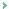The so called complicated concepts of square, multiplication of different numbers with various combinations have been explained in very simple and shortcut method. It has really been a great learning for me. This had certainly sharpened my calculation abilities and created interested in the subject. Thanks for all your great efforts in explaining the concept simple and precise so that a layman can understand the concept without much difficulty.

VENU GOPAL NOUDURI
11/04/2018 00:31 AM

Name *
Email ID
(will not be published)
Comment *
Characters
Verification Code*
Please fill the above code for verification.

Top | Education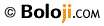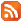RSS Feed Home | Privacy Policy | Disclaimer | Site Map